# 幻想小勇士破解版 v1.3.0内购版

• 详情
• 相关
• 评论
•••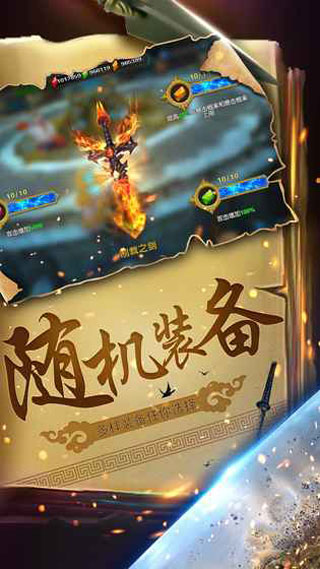••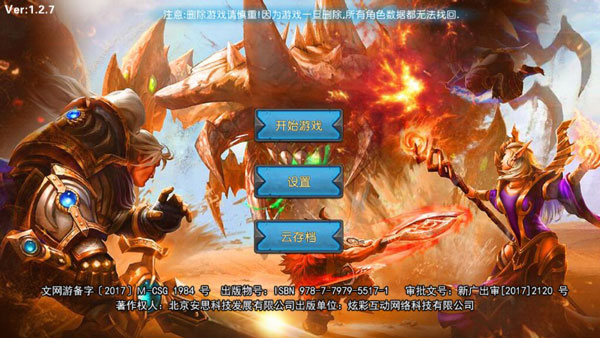1、英雄

2、专属武器

3、众多玩法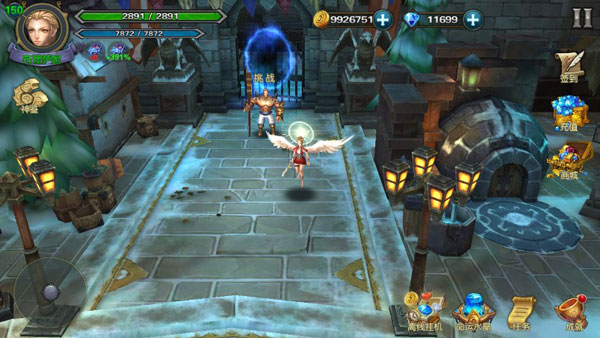【符文组合】
5+6+2装备属性+10%
5+5+3佣兵星级上限+1
5+1+7物品掉落概率10%
5+4+6挑战地图尝试次数+1
5+7+6亡灵掉落专属物品
6+5+8装备改造的消耗降低50%
6+4+6符文商人的符文等级上限+1
6+2+7战场出现红色奖励怪物
6+3+9虫族掉落专属物品
7+2+4装备属性+10%
7+4+5专属武器吸收灵魂
7+8+2背包容量+8
7+5+7物品掉落概率+10%
7+8+9恶魔掉落专属物品
7+10+3重置永恒+1
8+5+4每日任务经验+30%
8+7+4佣兵星级上限+1
8+6+3背包容量+8
8+1+8物品掉落概率+10%
8+9+3神秘商人允许刷新次数+1
8+6+10龙族掉落专属物品
9+4+7装备属性+10%
9+2+9试炼之塔奖励增加
9+8+5背包容量+8
1+1+1所有技能等级+1
1+3+2装备属性+10%
1+2+4每日任务经验+10%
1+3+3雪宝会给你讲笑话
1+4+3宝石合成成功率永久提高5%
1+2+5人族掉落专属物品
2+3+4背包容量+8
2+1+3符文商人的符文等级上限+1
2+2+2佣兵星级上限+1
2+1+4试炼之塔奖励增加
2+4+5物品掉落概率+10%
2+5+1重置永恒+1
2+6+4精灵掉落专属物品
3+1+2大转盘水晶消耗-1
3+2+3装备属性+10%
3+3+5每日任务水晶币奖励+1
3+4+4佣兵星级上限+1
3+5+5物品掉落概率+10%
3+7+2矮人掉落专属物品
4+2+1试炼之塔奖励增加
4+5+2每日任务经验+20%
4+1+6背包容量+8
4+2+7神秘商人允许刷新次数+1
4+5+8兽人掉落专属物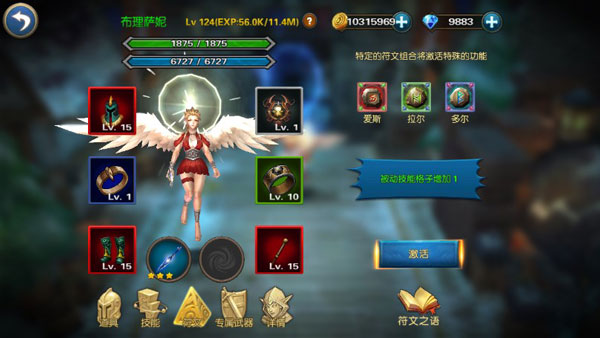3个属性：生命加成，防御加成，生命恢复加成。
3属性最高级别都是120级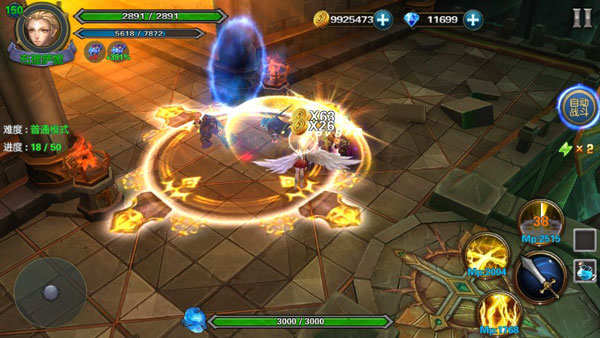120级的属性：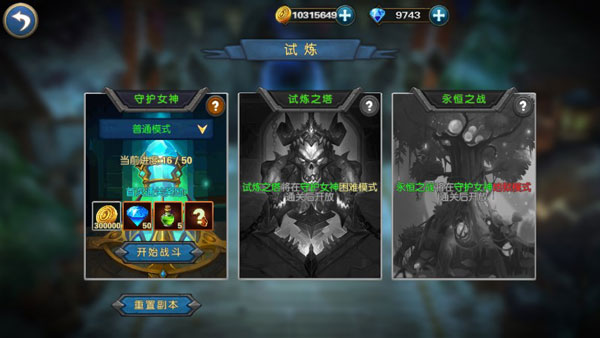【推荐】
1、智力英雄

2、敏捷英雄

3、力量英雄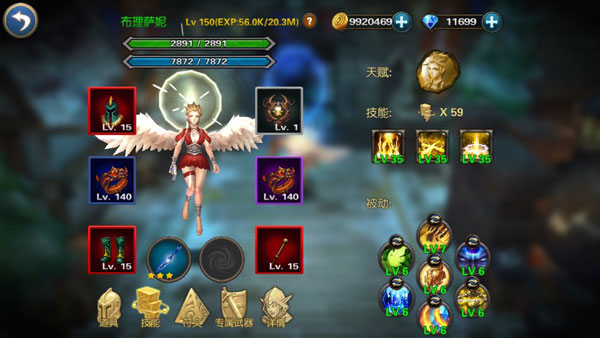0条评论

大家还喜欢这些：

+